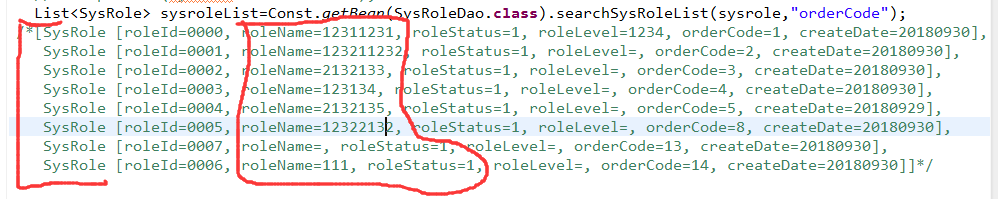list与数组之间的转换，一口老血在心中。1.注释部分都是sysroleList的输出值，现在想建立一个A[ ]数组，A[ ]存放的是sysroleList某一列的值
2现在有一个7，判断7是否存在于这个数组中。

5个回答

``````
for循环不就行了。
String []arr=new String[sysroleList.size()]；
for(int i=0;i<sysroleList.size();i++)
{
arr[i]=sysroleList.get(i).getRoleName();  //要什么数据就取什么数据，这里取了roleName数据
}

for(j=0;j<arr.length;j++){

if(arr[j].equals("7"));  //用包含也行，看你自己需求。
}
````````````int n = sysroleList.size()
String[] strs = new String[n];
for(int i = 0; i < n; i++){
strs[i] = sysroleList.get(i).getRoleName();
}

Arrays.asList(strs).contains("7");
``````

``````        // 解决方法1
List<String> aList = new ArrayList<>();
for (SysRole sysRole : sysroleList) {
}
// 判断7是否存在
String value = "7";
boolean flag = aList.contains(value);
System.err.println(flag ? "存在" : "不存在");

// 解决方法2 重写 SysRole的比较方法 直接通过你这个查询出来的sysroleList 判断
如果理解错了您的需求 请手动加我qq咨询  892042158

``````
`````` public class TestArrayList<T> {
public T[] toArray(List<T> list) {
if (list != null) {
T[] arr = (T[]) Array.newInstance(list.get(0).getClass(), list.size());
return (T[]) list.toArray(arr);
}
return null;
}

public boolean contain(T[] arr, T target, Comparator c) {
if (arr == null || arr.length == 0) {
return false;
}
for (T v : arr) {
if (c.isEqal(v, target)) {
return true;
}
}
return false;
}

public interface Comparator<T> {
public boolean isEqal(T a, T b);
}

public static void main(String[] args) {//测试
TestArrayList<String> util = new TestArrayList<String>();
List<String> valList = new ArrayList<String>();
System.out.println("list: " + valList);
String[] valArray = util.toArray(valList);
System.out.println("array: " + Arrays.toString(valArray));
//比较
boolean contain = util.contain(valArray, "222", new Comparator<String>() {

@Override
public boolean isEqal(String a, String b) {
return a != null && a.equals(b);
}
});
System.out.println(contain);
}
}

/*结果：
list: [111, 222]
array: [111, 222]
true

*/
``````